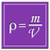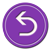# National Aeronautics and Space Administration

## Goddard Space Flight Center# Using the relationship between density, mass and volumeRecap: Your astronomy professor has tasked the class with determining the mass of the black hole candidate, Cygnus X-1. You thought of three possible ways to do this, one of which will give you the right answer. You've decided to try using the relationship between density, mass and volume.

You learned in science class that density is the amount of matter in a given volume. Density is a characteristic property of matter, which means that density can be used to identify a sample of matter and the size of the sample will not change the result. Density is determined by dividing the mass of a substance by the volume occupied by that mass.

So, if you know the volume and density of an object, you can find the mass by simply rearranging the above equation.Use this technique to solve for Cyg X-1's massReturn to the beginning and try another approach